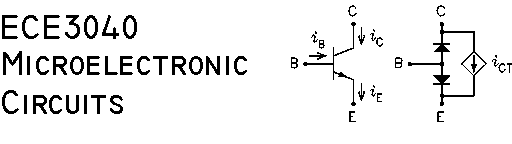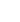### Cell Phone Policy

Answers to selected problems in the text by Jaeger and Blalock are at the url: http://www.jaegerblalock.com/MCD_2E_Answers.pdf

### Summer 2005 Class Documents

Syllabus

#### Class Notes

• Set 01
Conduction in Semiconductors
Classification of Conductors
Conduction in Metals - Drift Velocity, Charge Density, Current Density, Conductivity, Resistance
Conduction in Intrinsic Semiconductors - Recombinations, Intrinsic Concentration, Conductivity
n-Type and p-Type Semiconductors - The n-Type Semiconductor, The p-Type Semiconductor, Mass-Action Law, Electrical Neutrality, Conductivity, Diffusion Current, Total Current
The Open-Circuited p-n Junction - Depletion Region, Built-In Potential
The Short-Circuited p-n Junction
The Biased p-n Junction - Reverse Bias, Forward Bias
• Set 02
The Junction Diode - Terminal Characteristics of the Diode, Temperature Dependence of the Diode Current, Graphical Solution of Diode Circuits, A Piecewise Linear Diode Model, The Diode Small-Signal Resistance, Breakdown Characteristics, Charge Storage in the Diode, The Diode Small-Signal Capacitance, The Schottky Barrier Diode, The SPICE Diode Model
• Set 03
Diode Clipper Circuits, The Boost dc-to-dc Converter, The Photo Diode, The Light Emitting Diode, The Enhancement Mode MOSFET
• Set 04
The MOSFET Bias Equation, The Depletion Mode MOSFET, p-Channel MOSFETS, The Junction Field Effect Transistor, The Small-Signal Pi Model of the MOSFET, The Small-Signal T Model of the MOSFET
• Set 05
Modeling the MOSFET Body Effect, The Common-Source Amplifier, The Common-Drain Amplifier, The Common-Gate Amplifier
• Set 06
The MOSFET Differential Amplifier, The Bipolar Junction Transistor (BJT), The BJT Bias Equation
• Set 07
The Small-Signal Pi Model of the BJT, The Small-Signal T Model of the BJT, The Common-Collector Amplifier, The Common-Base Amplifier, A CE/CC Example, The Simplified T Model of the BJT
• Set 08
The BJT Differential Amplifier, The Complimentary Common-Collector Amplifier
• Set 09
• Part One
Model of the BJT Operational Amplifier
• Part Two
Ideal Op-Amp Circuits
• Part Three
Transfer Functions and Bode Plots, Impedance Transfer Functions, Voltage Divider Transfer Functions
• Set 10
CMOS Logic Gates, Noise Margins, The CMOS NOR Gate, The CMOS NAND Gate, The BJT TTL NAND GateThis page is not a publication of the Georgia Institute of Technology and the Georgia Institute of Technology has not edited or examined the content. The author of this page is solely responsible for the content.